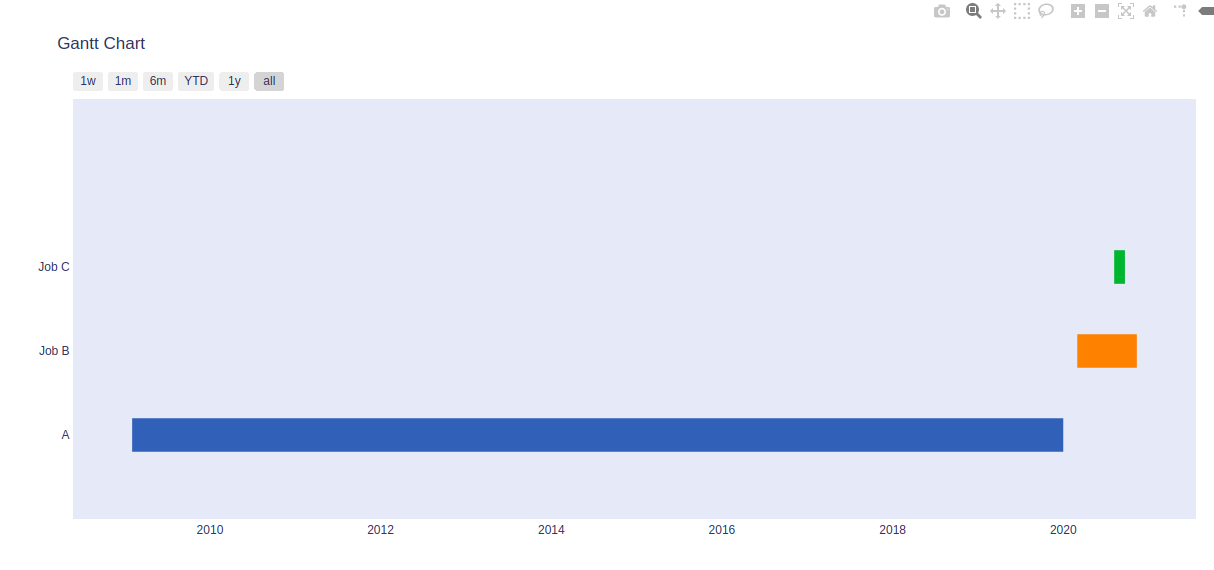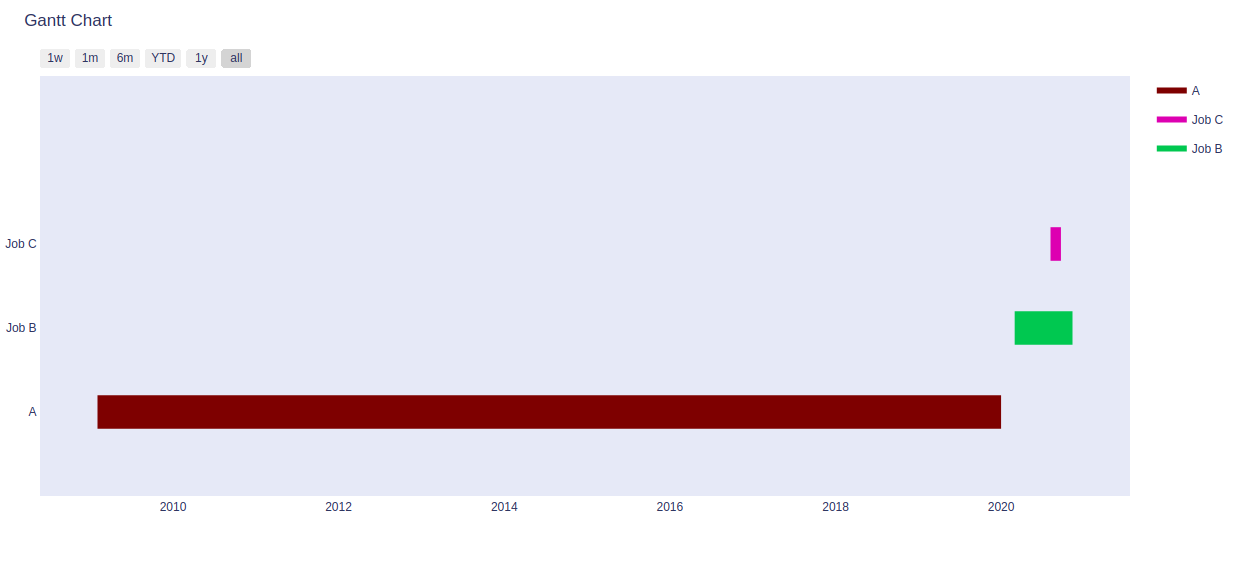# Gantt Chart in plotly

• Last Updated : 29 Aug, 2020

Plotly is a Python library that is used to design graphs, especially interactive graphs. It can plot various graphs and charts like histogram, barplot, boxplot, spreadplot, and many more. It is mainly used in data analysis as well as financial analysis. plotly is an interactive visualization library.

## Gantt Chart

A Gantt chart is parallel to bar chart that delineates a project schedule. In Gantt chart tasks are performed in the vertical axis and the time intervals are performed in the horizontal axis. The width of the horizontal bars in the graph shows the endurance of both the activity. A  Gantt chart is made up of numerous different elements. the main 8 key components are:

• Timeline
• Date line
• Bars
• Milestones
• Dependencies
• Progress
• Resource assigned

Syntax: plotly.figure_factory.create_gantt(df, colors=None, index_col=None, show_colorbar=False, reverse_colors=False, title=’Gantt Chart’, bar_width=0.2, showgrid_x=False, showgrid_y=False, height=600, width=None, tasks=None, task_names=None, data=None, group_tasks=False, show_hover_fill=True)

Parameters:

df: input data for gantt chart. Must be either a a dataframe or a list.

colors:  either a plotly scale name, an rgb or hex color, a color tuple or a list of colors

show_colorbar: determines if colorbar will be visible. Only applies if values in the index column are numeric.

show_hover_fill: enables/disables the hovertext for the filled area of the chart.

title: title of the chart

height: Height of the chart

weight: Weight of the chart

Example 1:

## Python3

 `import` `plotly.figure_factory as ff ` ` `  `df ``=` `[``dict``(Task``=``"A"``, Start``=``'2020-01-01'``, Finish``=``'2009-02-02'``), ` `      ``dict``(Task``=``"Job B"``, Start``=``'2020-03-01'``, Finish``=``'2020-11-11'``), ` `      ``dict``(Task``=``"Job C"``, Start``=``'2020-08-06'``, Finish``=``'2020-09-21'``)] ` ` `  `fig ``=` `ff.create_gantt(df) ` `fig.show()`

Output:Example 2:

## Python3

 `import` `plotly.figure_factory as ff ` ` `  `df ``=` `[``dict``(Task``=``"A"``, Start``=``'2020-01-01'``, Finish``=``'2009-02-02'``), ` `      ``dict``(Task``=``"Job B"``, Start``=``'2020-03-01'``, Finish``=``'2020-11-11'``), ` `      ``dict``(Task``=``"Job C"``, Start``=``'2020-08-06'``, Finish``=``'2020-09-21'``)] ` ` `  `colors ``=` `[``'#7a0504'``, (``0.2``, ``0.7``, ``0.3``), ``'rgb(210, 60, 180)'``] ` ` `  `fig ``=` `ff.create_gantt(df, show_colorbar ``=` `True``, colors ``=` `colors    ) ` `fig.show()`

Output:My Personal Notes arrow_drop_up
Recommended Articles
Page :